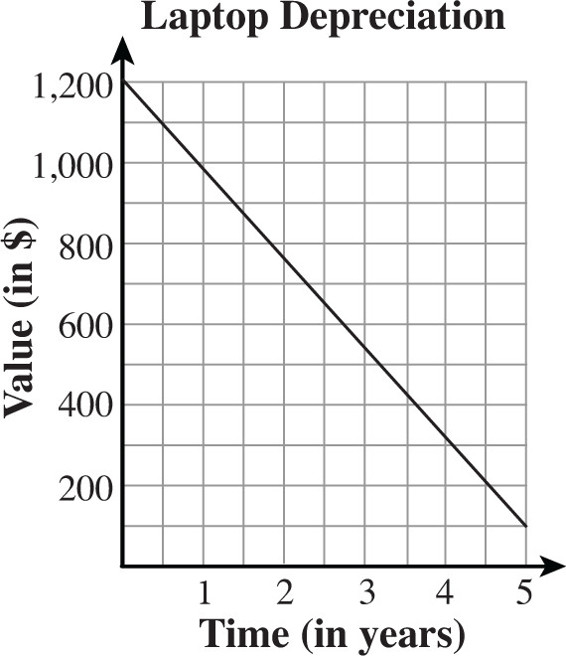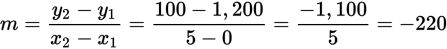# SAT Math Multiple Choice Question 308: Answer and Explanation

### Test Information

Question: 308

8.The figure above shows the straight-line depreciation of a laptop computer over the first five years of its use. According to the figure, what is the average rate of change in dollars per year of the value of the computer over the five-year period?

• A. -1,100
• B. -220
• C. -100
• D. 100

Explanation:

B

Difficulty: Medium

Category: Heart of Algebra / Linear Equations

Strategic Advice: Average rate of change is the same as slope, so use the slope formula.

Getting to the Answer: To find the average rate of change over the 5-year period, find the slope between the starting point (0, 1,200) and the ending point (5, 100).The average rate of change is negative because the laptop decreases in value over time.

Note: Because the question involves straight-line depreciation, you could have used any two points on the graph to find the slope. As a general rule, however, you should use the endpoints of the given time interval.